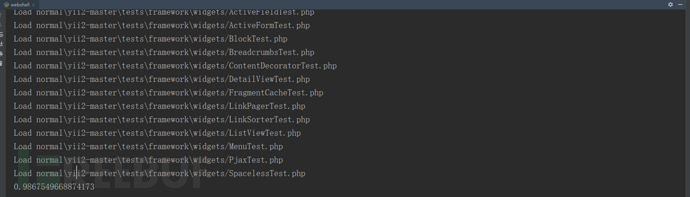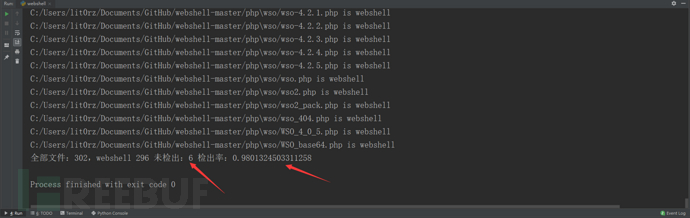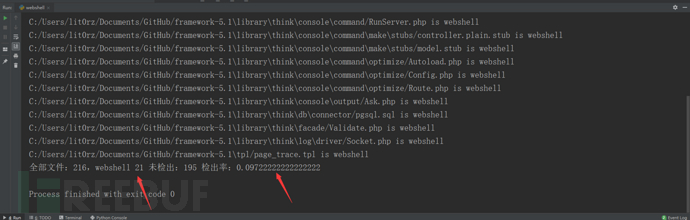# 机器学习入门之像使用Print一样使用算法检测WebShell

## 0×00 pre

https://github.com/zltningx/webshellgg

## 0×01 准备样本

https://github.com/tennc/webshell

https://github.com/JohnTroony/php-webshells

https://github.com/ysrc/webshell-sample

https://github.com/tanjiti/webshellSample

https://github.com/xl7dev/WebShell

https://github.com/WordPress/WordPress

https://github.com/typecho/typecho

https://github.com/bcit-ci/CodeIgniter

https://github.com/laravel/laravel

https://github.com/symfony/symfony

## 0×02 实现

``pip install sklearn``

### step1： 特征提取

t = b”

with open(file_path, “rb”) as f:

for line in f:

line = line.strip(b’\r\n’)

t += line

return t

files_list = []

for r, d, files in os.walk(path):

for file in files:

if file.endswith(‘.php’): #过滤非php文件

file_path = r + ‘/’ + file

files_list.append(t)

return files_list

``webshell_files = load_files(webshell_dir)``

``wp_files = load_files(normal_dir)``

black_count = len(webshell_files)

white_count = len(wp_files)

y1 = *black_count

y2 = *white_count

x = webshell_files + wp_files

y = y1 + y2

cv = CountVectorizer(ngram_range=(2, 2), decode_error=“ignore”,

token_pattern=r’\b\w+\b’, min_df=1,

max_df=1.0, max_features=max_features)

x = cv.fit_transform(x).toarray()

transformer = TfidfTransformer(smooth_idf=False)

x = transformer.fit_transform(x).toarray()

### step2： MLP算法

clf = MLPClassifier(solver=“lbfgs”, alpha=1e-5, hidden_layer_sizes=(5, 2),

random_state=1)

x_train, x_test, y_train, y_test = train_test_split(x, y, test_size=0.4, random_state=0)

clf.fit(x_train, y_train)

``print(np.mean(y_test==clf.predict(x_test)))``### step3： 编写检测函数

def check(clf, cv, transformer, path):

all = 0

webshell = 0

not_webshell = 0

for r, d, files in os.walk(path):

for file in files:

file_path = r + ‘/’ + file

t_list = list()

t_list.append(t)

x = cv.transform(t_list).toarray()

x = transformer.transform(x).toarray()

y_pred = clf.predict(x)

all += 1

if y_pred == 1:

print(“{} is webshell”.format(file_path))

webshell += 1

else:

not_webshell += 1

print(“全部文件：{}，webshell {} 未检出：{} 检出率：{}”.format(all, webshell, not_webshell, webshell/all))

x = cv.transform(t_list).toarray()

x = transformer.transform(x).toarray()### step4: 持久化

``from sklearn.externals import joblib``

``import pickle``

MLPClassifier对象

CountVectorizer对象

TfidfTransformer对象

joblib.dump(clf, “mlp.pkl“)

joblib.dump(cv, “cv.pkl“)

joblib.dump(transformer, “transformer.pkl“)

``joblib.load("mlp.pkl")``

## 0×03 END

*本文原创作者：littt0，本文属FreeBuf原创奖励计划，未经许可禁止转载